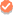Home > Multiply Decimals Games# Multiply Decimals Games for Kids

Grade 5 math introduces complex topics like decimal arithmetic. After decimal addition & subtraction, let SplashLearn's online games help your kids with decimal multiplication activities starting with multiplying with the power of 10, whole numbers, & finally, multiplication of two decimal numbers... Read more

•Personalized Learning
•Fun Rewards
•Actionable Reports